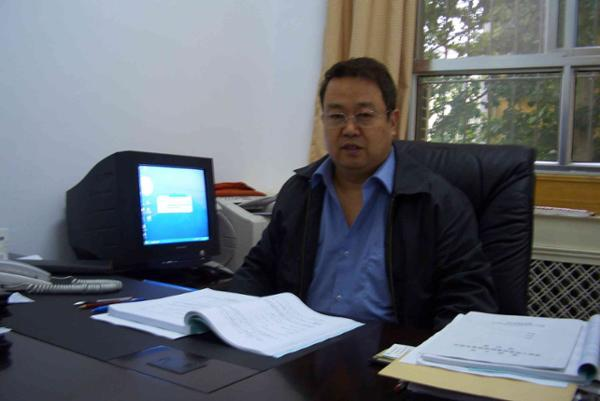郑大考研服务站分数线# 耿献国教授E-mail：xggeng@zzu.edu.cn

1．X.G. Geng, Y.Y. Zhai and H.H. Dai, Algebro-geometric solutions of the coupled modified Korteweg-de Vries hierarchy, Advances in Mathematics 263 (2014) 123-153.

2．G.L. He, X.G. Geng, L.H. Wu, Algebro-geometric quasi-periodic solutions to the three-wave resonant interaction hierarchy, SIAM Journal on Mathematical Analysis 46, 2 (2014) 1348-1384.

3．X.G. Geng, LH. Wu and G.L. He, Quasi-periodic solutions of the Kaup-Kupershmidt hierarchy, Journal of Nonlinear Science 23, 4 (2013)  527-555.

4．X.G. Geng and H. Wang, Coupled Camassa-Holm equations, N-peakons and infinitely many conservation laws, Journal of Mathematical Analysis and Applications 403, 1 (2013) 262-271.

5．X.G. Geng, B. Xue, and LH. Wu, A super Camassa–Holm equation with N-peakon solutions, Studies in Applied Mathematics 130, 1 (2013) 1-16.

6．X.G. Geng and D. Gong, Quasi-periodic solutions of the discrete mKdV hierarchy, International Journal of Geometric Methods in Modern Physics 10, 3 (2013) 1250094.

7．X.G. Geng and Y.Y. Lv, Darboux transformation for an integrable generalization of the nonlinear Schrodinger equation, Nonlinear Dynamics 69, 4 (2012) 1621-1630.

8．L.H. Wu, G.L. He and X.G. Geng, Quasi-periodic solutions to the two-component nonlinear Klein–Gordon equation, Journal of Geometry and Physics 66 (2013) 1-17.

9．X.G. Geng, L.H. Wu and G.L. He, Algebro-geometric constructions of the modified Boussinesq flows and quasi-periodic solutions, Physica D：Nonlinear Phenomena 240, 16 (2011) 1262–1288.

10．X.G. Geng and B. Xue, A three-component generalization of Camassa-Holm equation with N-peakon solutions, Advances in Mathematics 226, 1 (2011) 827-839.

11．X.G. Geng and B. Xue, Quasi-periodic solutions of mixed AKNS equations, Nonlinear Analysis: Theory, Methods & Applications 73, 11 (2010) 3662-3674.

12．X.G. Geng and B. Xue, Soliton solutions and quasiperiodic solutions of modified Korteweg-de Vries type equations, Journal of Mathematical Physics 51, 6 (2010) 063516.

13．X.G. Geng and L.H. Wu, A new super-extension of the KdV hierarchy, Applied Mathematics Letter 23, 6 (2010) 716–721.

14．X.G. Geng and B. Xue, An extension of integrable peakon equations with cubic nonlinearity, Nonlinearity 22, 8 (2009) 1847–1856.

15．X.G. Geng, H.F. Ren and G.L. He, Darboux transformation for a generalized Hirota-Satsuma coupled Korteweg–de Vries equation, Physical Review E 79, 5 (2009) 056602.

16．X.G. Geng, H.H. Dai and J.Y. Zhu, Decomposition of the discrete Ablowitz- Ladik hierarchy, Studies in Applied Mathematics 118, 3 (2007) 281-312.

17．X.G. Geng and T. Su, Discrete coupled derivative nonlinear Schrodinger equations and their quasi-periodic solutions, Journal of Physics A: Math. Theor. 40, 3 (2007) 433-453.

18．X.G. Geng and H.H. Dai, Nonlinearization of the Lax pairs for discrete Ablowitz–Ladik hierarchy, Journal of Mathematical Analysis and Applications 327, 2 (2007) 829-853.

19．X.G. Geng, H.H. Dai and C.W. Cao, Algebro–geometric constructions of the discrete Ablowitz–Ladik flows and applications, Journal of Mathematical Physics 44, 10 (2003) 4573-4588.

20．X.G. Geng, Algebraic-geometrical solutions of some multidimensional nonlinear evolution equations, Journal of Physics A: Math. Gen. 36, 9 (2003) 2289-2303.